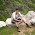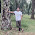## January 18, 2014

### Problem: Sequential Element

Another problem for you!

Consider the following circuit. Assume that each inverter takes 1 time unit for a low-to-high or high-to-low transition. Assume that it takes 1 time unit for a pull-up path or pull-down path to pull-up or pull-down respectively. Ignore any leakage effects. Assume VDD >> VT. Also assume that there is no skew between CLK and CLK_BAR and assume that rise/fall times on all signals are zero.

1. What type of sequential element is the above circuit? (Hint: Plotting the X, Y and Q with respect to the waveform of CLK and D might help you in finding the answer.)
2. What is the propagation delay of this sequential element?

Source:
Massachusetts Institute of Technology
Dept. of Electrical Engineering and Computer Sciences
Analysis and Design of Digital Integrated Circuits- Fall 2003, Quiz #2
Prof. Anantha Chandrakasan

#### 5 comments:

1.Seems to be a dual edge triggered flop with inverted output.

2.Yes this is a dual edge triggered ff. For the delay, pull up and pull downs are not obvious. Delay is 1 unit

1.I had solved this problem long time back and I remember it to be a dual edge triggered flip-flop. However, I do not remember the propagation delay. I'll try to solve it again and then report my answer.

Thanks for posting! :)

3.This circuit is sampling the D input on both positive and negative transitions of the clock (dual edge triggered) with a clock to the output delay of 2 time units.

4.If I read the circuit correctly, then after sampling the Q state in the FF is stored by the charges on the gates of the output FETs. So this will not stay there forever. However, if the D input changes during this "hold" phase, the Q output will be driven to a high impedance "Z", no?
So I'd not call this a FlipFlop at all - or where is my misunderstanding?